# Spatial analysis of NYC Shootings using the SPPT

As a follow up to my prior post on spatial sample size recommendations for the SPPT test, I figured I would show an actual analysis of spatial changes in crime. I’ve previously written about how NYC shootings appear to be going up by a similar amount in each precinct. We can do a similar analysis, but at smaller geographic spatial units, to see if that holds true for everywhere.

The data and R code to follow along can be downloaded here. But I will copy-paste below to walk you through.

So first I load in the libraries I will be using and set my working directory:

``````###################################################
library(sppt)
library(sp)
library(raster)
library(rgdal)
library(rgeos)

my_dir <- 'C:\\Users\\andre\\OneDrive\\Desktop\\NYC_Shootings_SPPT'
setwd(my_dir)
###################################################
``````

Now we just need to do alittle data prep for the NYC data. Concat the old and new files, convert the data fields for some of the info, and do some date manipulation. I choose the pre/post date here March 1st 2020, but also note we had the Floyd protests not to long after (so calling these Covid vs protest increases is pretty much confounded).

``````###################################################
# Read in the shooting data

# Just one column off
print( cbind(names(old_shoot), names(new_shoot)) )
names(new_shoot) <- names(old_shoot)
shooting <- rbind(old_shoot,new_shoot)

# I need to conver the coordinates to numeric fields
# and the dates to a date field

coord_fields <- c('X_COORD_CD','Y_COORD_CD')
for (c in coord_fields){
shooting[,c] <- as.numeric(gsub(",","",shooting[,c])) #replacing commas in 2018 data
}

# How many per year to check no funny business
table(substring(shooting\$OCCUR_DATE,7,10))

# Making a datetime variable in R
shooting\$OCCUR_DATE <- as.Date(shooting\$OCCUR_DATE, format = "%m/%d/%Y", tz = "America/New_York")

# Making a post date to split after Covid started
begin_date <- as.Date('03/01/2020', format="%m/%d/%Y")
shooting\$Pre <- ifelse(shooting\$OCCUR_DATE < begin_date,1,0)

#There is no missing data
summary(shooting)
###################################################
``````

Next I read in a shapefile of the census tracts for NYC. (Pro-tip for NYC GIS data, I like to use Bytes of the Big Apple where available.) The interior has a few dongles (probably for here should have started with a borough outline file), so I do a tiny buffer to get rid of those interior dongles, and then smooth the polygon slightly. To check and make sure my crime data lines up, I superimpose with a tiny dot map — this is also a great/simple way to see the overall shooting density without the hassle of other types of hot spot maps.

``````###################################################
# Read in the census tract data

summary(nyc_ct)
plot(nyc_ct)
nrow(nyc_ct) #2165 tracts

# Dissolve to a citywide file
nyc_ct\$const <- 1
nyc_outline <- gUnaryUnion(nyc_ct, id = nyc_ct\$const)
plot(nyc_outline)

# Area in square feet
total_area <- area(nyc_outline)
# 8423930027

# Turning crimes into spatial point data frame
coordinates(shooting) <- coord_fields
crs(shooting) <- crs(nyc_ct)

# This gets rid of a few dongles in the interior
nyc_buff <- gBuffer(nyc_outline,1,byid=FALSE)
nyc_simpler <- gSimplify(nyc_buff, 500, topologyPreserve=FALSE)

# Checking to make sure everything lines up
png('NYC_Shootings.png',units='in',res=1000,height=6,width=6,type='cairo')
plot(nyc_simpler)
points(coordinates(shooting),pch='.')
dev.off()
###################################################
``````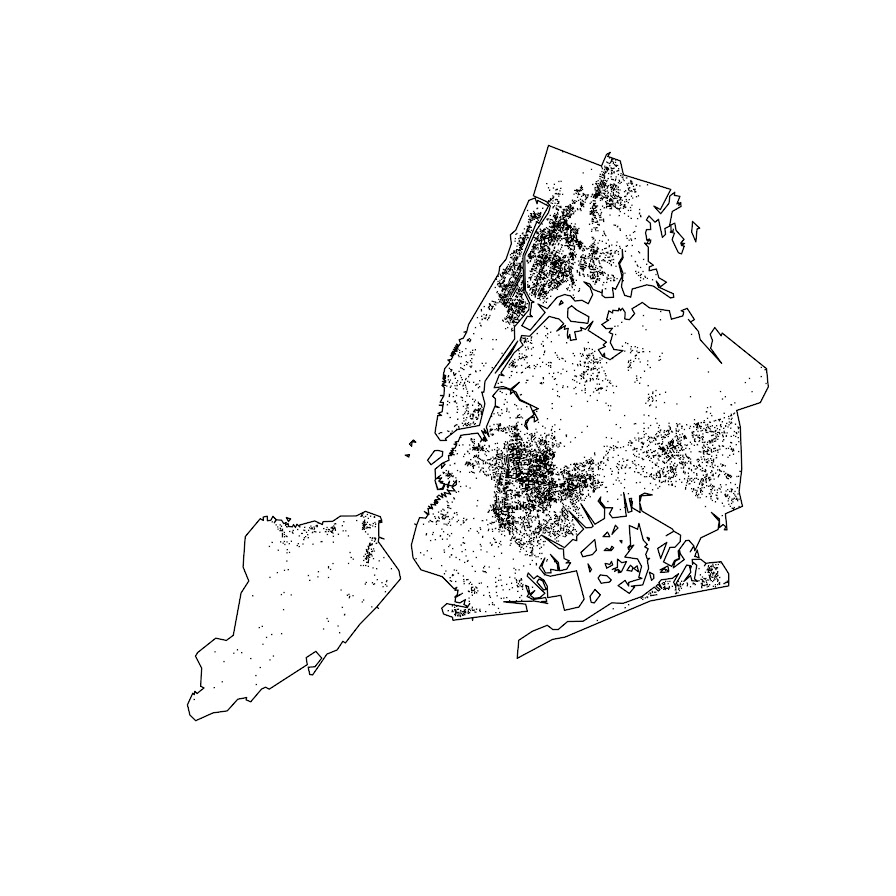The next part I created a function to generate a nice grid over an outline area of your choice to do the SPPT analysis. What this does is generates the regular grid, turns it from a raster to a vector polygon format, and then filters out polygons with 0 overlapping crimes (so in the subsequent SPPT test these areas will all be 0% vs 0%, so not much point in checking them for differences over time!).

You can see the logic from the prior blog post, if I want to use the area with power to detect big changes, I want `N*0.85`. Since I am comparing data over 10 years compared to 1+ years, they are big differences, so I treat N here as 1.5 times the newer dataset, which ends up being around a suggested 3,141 spatial units. Given the area for the overall NYC, this translates to grid cells that are about 1600 by 1600 feet. Once I select out all the 0 grid cells, there only ends up being a total of 1,655 grid cells for the final SPPT analysis.

``````###################################################
# Function to create sppt grid over areas with
# Observed crimes

grid_crimes <- function(outline,crimes,size){
# First creating a raster given the outline extent
base_raster <- raster(ext = extent(outline), res=size)
projection(base_raster) <- crs(outline)
# Getting the coverage for a grid cell over the city area
# Turning into a polygon
base_poly <- rasterToPolygons(base_raster,dissolve=FALSE)
xy_df <- as.data.frame(base_raster,long=T,xy=T)
base_poly\$x <- xy_df\$x
base_poly\$y <- xy_df\$y
base_poly\$poly_id <- 1:nrow(base_poly)
# May also want to select based on layer value
# sel_poly <- base_poly[base_poly\$layer > 0.05,]
# means the grid cell has more than 5% in the outline area
# Selecting only grid cells with an observed crime
ov_crime <- over(crimes,base_poly)
any_crime <- unique(ov_crime\$poly_id)
sub_poly <- base_poly[base_poly\$poly_id %in% any_crime,]
# Redo the id
sub_poly\$poly_id <- 1:nrow(sub_poly)
return(sub_poly)
}

# Calculating suggested sample size
total_counts <- as.data.frame(table(shooting\$Pre))
print(total_counts)

# Lets go with the pre-total times 1.5
total_n <- total_counts\$Freq*1.5

# Figure out the total number of grid cells
# Given the total area
side <- sqrt( total_area/total_n )
print(side)
# 1637, lets just round down to 1600

poly_cells <- grid_crimes(nyc_simpler,shooting,1600)
print(nrow(poly_cells)) #1655

png('NYC_GridCells.png',units='in',res=1000,height=6,width=6,type='cairo')
plot(nyc_simpler)
dev.off()
###################################################
``````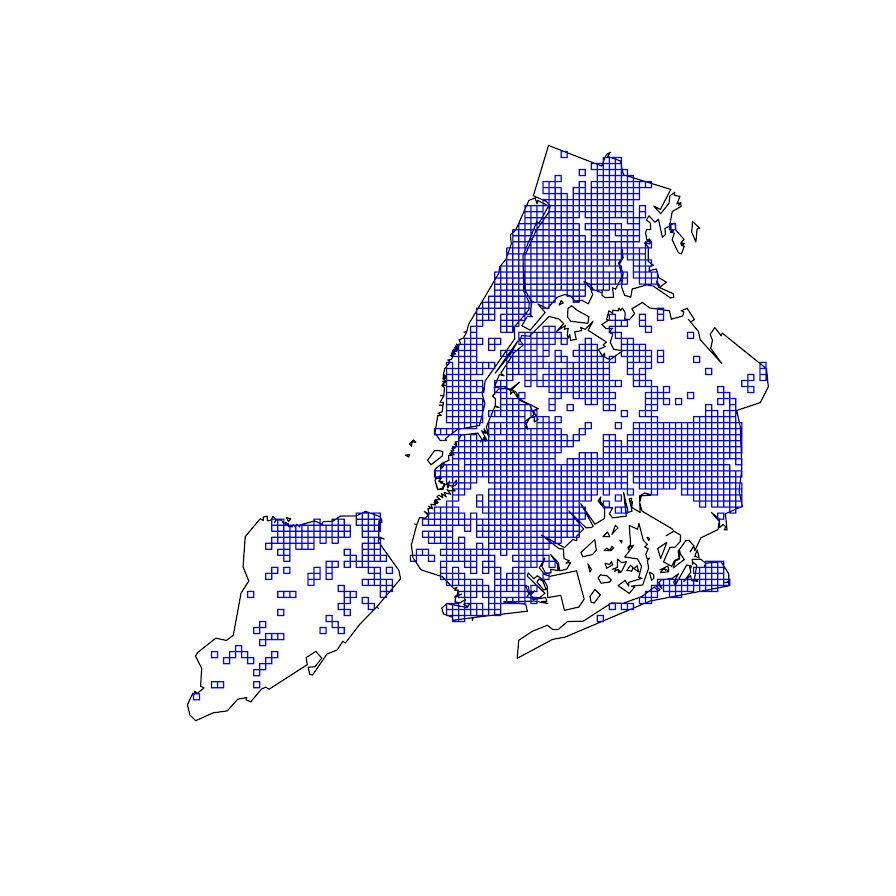Next part is to split the data into pre/post, and do the SPPT analysis. Here I use all the defaults, the Chi-square test for proportional differences, along with a correction for multiple comparisons. Without the multiple comparison correction, we have a total of 174 grid cells that have a p-value < 0.05 for the differences in proportions for an S index of around 89%. With the multiple comparison correction though, the majority of those p-values are adjusted to be above 0.05, and only 25 remain afterwards (98% S-index). You can see in the screenshot that all of those significant differences are increases in proportions from the pre to post. While a few are 0 shootings to a handful of shootings (suggesting diffusion), the majority are areas that had multiple shootings in the historical data, they are just at a higher intensity now.

``````###################################################
# Now lets do the sppt analysis

split_shoot <- split(shooting,shooting\$Pre)
pre <- split_shoot\$`1`
post <- split_shoot\$`0`

library(dplyr)
sppt_diff <- sppt_diff(pre, post, poly_cells)
summary(sppt_diff)

sum(sppt_diff\$p.value < 0.05) #174, around 89% similarity
sum(sppt_diff\$p.adjusted  < 0.05) #25, 98% similarity

# Lets select out the increases/decreases
# And just map those

sppt_sig <- sppt_diff[sig,]
head(sppt_sig,25) # to check out all increases
###################################################
``````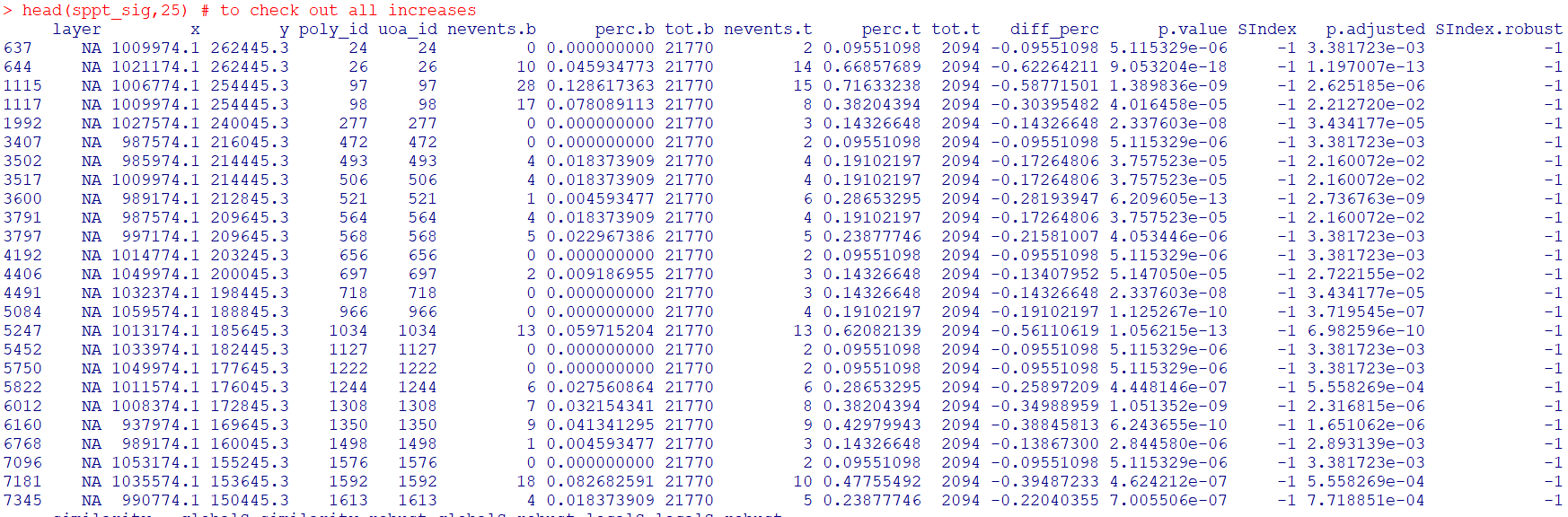The table is not all that helpful though for really digging into patterns, we need to map out the differences. The first here is a map showing the significant grid cells. They are somewhat tiny though, so you have to kind of look close to see where they are. The second map uses proportional circles to the percent difference (so bigger circles show larger increases). I am too lazy to do a legend/scale, but see my prior post on a hexbin map, or the sp website in the comments.

``````###################################################
# Making a map
png('NYC_SigCells.png',units='in',res=1000,height=6,width=6,type='cairo')
plot(nyc_simpler,lwd=1.5)
dev.off()

circ_sizes <- sqrt(-sppt_sig\$diff_perc)*3

png('NYC_SigCircles.png',units='in',res=1000,height=6,width=6,type='cairo')
plot(nyc_simpler,lwd=1.5)
points(coordinates(sppt_sig),pch=21,cex=circ_sizes,bg='red')
dev.off()

# check out https://edzer.github.io/sp/
# For nicer maps/legends/etc.
###################################################
``````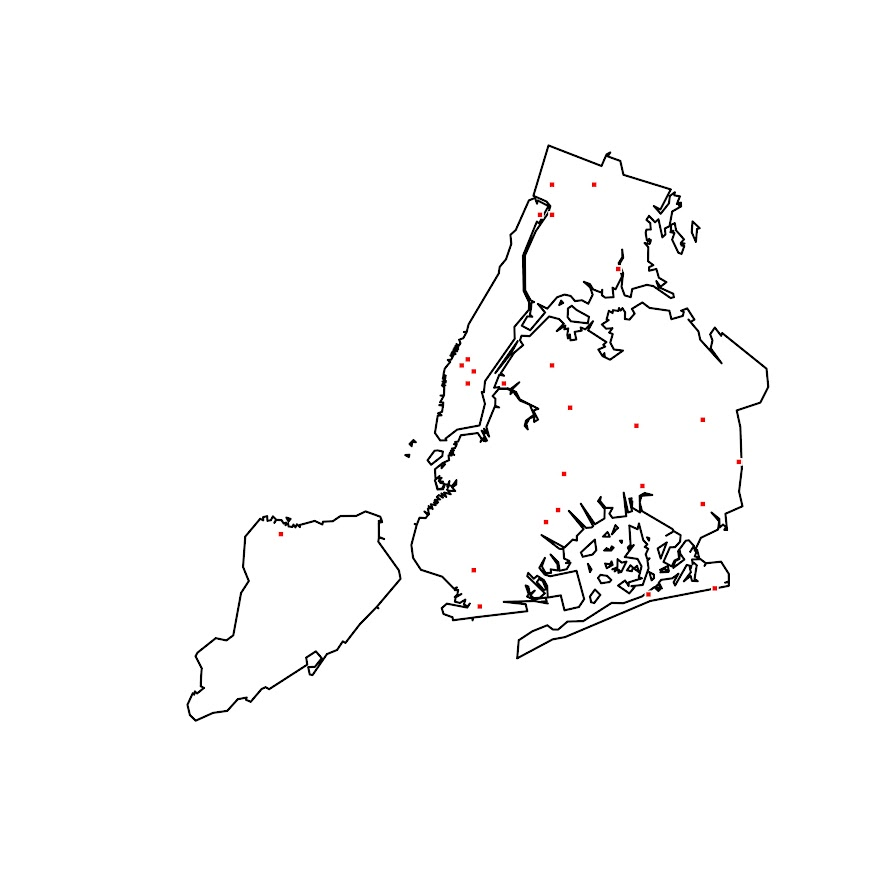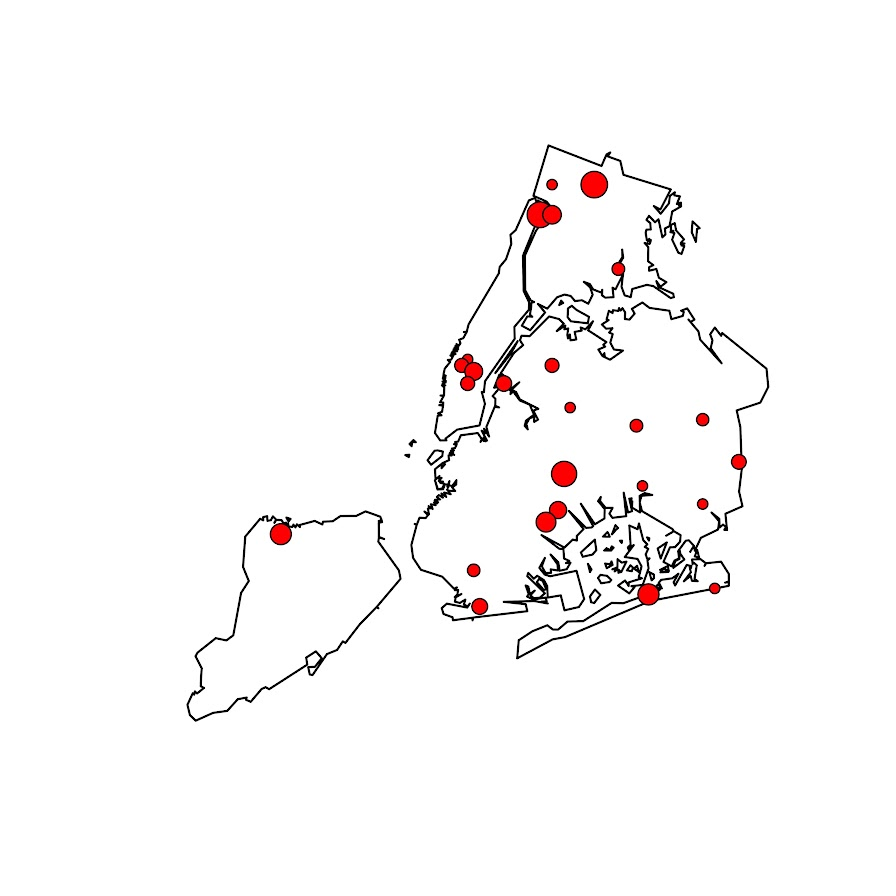So the increases appear pretty spread out. We have a few notable ones that made the news right in the thick of things in Manhattan, but there are examples of grid cells that increased scattered all over the boroughs. I am not going to the trouble here, but if I were a crime analyst working on this, I would export this to a format where I could zoom into the local areas and drill down into the specific incidents. You can do that either in ArcGIS, or more directly in R by creating a leaflet map.

So if folks have any better ideas for testing out crime increases I am all ears. At some point will give the R package sparr a try. (Here you could treat pre as the controls and post as the cases.) I am not a real big fan of over interpreting changes in kernel density estimates though (they can be quite noisy, and heavily influenced by the bandwidth), so I do like the SPPT analysis by default (but it swaps out a different problem with choosing a reasonable grid cell size).